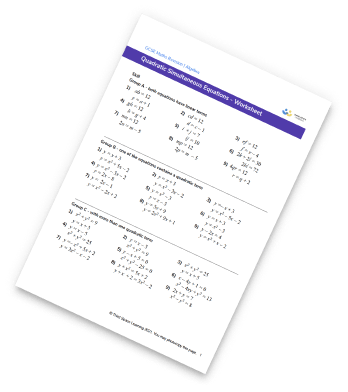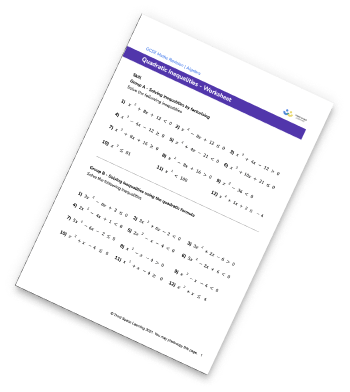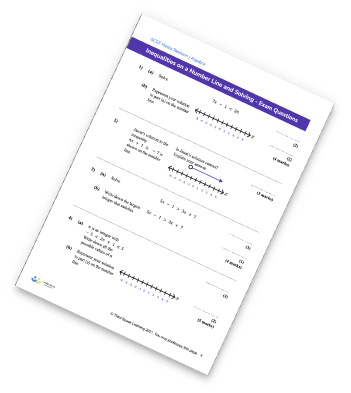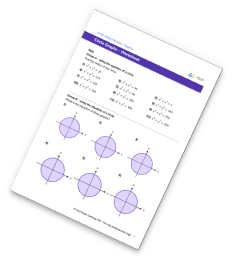# Simultaneous Equations Worksheet• This field is for validation purposes and should be left unchanged.

You can unsubscribe at any time (each email we send will contain an easy way to unsubscribe). To find out more about how we use your data, see our privacy policy.

• Section 1 of the simultaneous equations worksheet contains 20+ skills-based simultaneous equations questions, in 3 groups to support differentiation
• Section 2 contains 3 applied questions with a mix of worded problems and deeper problem solving questions
• Section 3 contains 3 foundation and higher level GCSE exam style questions to aid the practising of solving simultaneous equations
• Answers and a mark scheme for all questions are provided
• Questions follow variation theory with plenty of opportunities for students to work independently at their own level
• All questions created by fully qualified expert secondary maths teachers
• Suitable for GCSE maths revision for AQA, OCR and Edexcel exam boards

### Simultaneous equations at a glance

Simultaneous equations are pairs of equations with two unknowns. There are different types of simultaneous equations that we can solve. When both equations are linear equations we can use the elimination method. Here, we need to compare the coefficients of the variables and find ones that match. One or both of the equations will often need to be multiplied by an integer so that the coefficients of one of the variables is the same in both equations. The equations can then be added or subtracted to eliminate one of the variables. Once one of the variables is found, we can then substitute it back into one of the equations in order to find the second variable.

We can also solve linear simultaneous equations by using the substitution method where one of the equations is substituted into the other equation. We can then solve the equation to work out one of the variables and then substitute it back into one of the original equations to find the value of the second variable.

We can also solve a pair of simultaneous equations where the first equation is linear and the second equation is quadratic by using the substitution method. The linear equation is used to make a substitution into the nonlinear equation.

Solving simultaneous equations can also be used to find the point of intersection of straight lines and curved lines.

Looking forward, students can then progress to additional algebra worksheets, for example a simplifying expressions worksheets, simultaneous equations worksheet, or factorising worksheet.For more teaching and learning support on Algebra our GCSE maths lessons provide step by step support for all GCSE maths concepts.

## Related worksheetsInequalities On A Number Line WorksheetCircle Graph Worksheet## Do you have KS4 students who need more focused attention to succeed at GCSE?There will be students in your class who require individual attention to help them succeed in their maths GCSEs. In a class of 30, it’s not always easy to provide.

Help your students feel confident with exam-style questions and the strategies they’ll need to answer them correctly with our dedicated GCSE maths revision programme.

Lessons are selected to provide support where each student needs it most, and specially-trained GCSE maths tutors adapt the pitch and pace of each lesson. This ensures a personalised revision programme that raises grades and boosts confidence.

Find out more